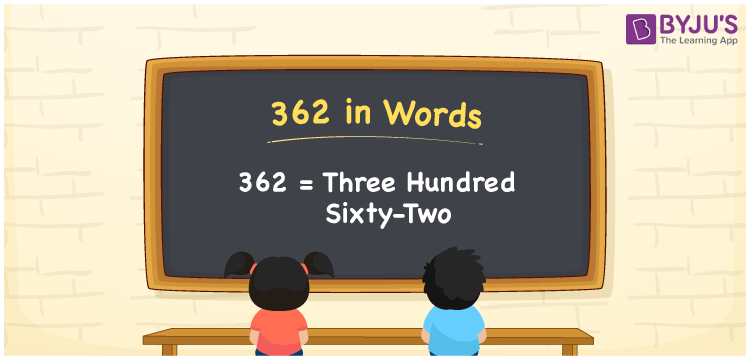# 362 in Words

362 in words is written as Three hundred sixty-two. In both the International System of Numerals and the Indian System of Numerals, 362 is written as Three hundred sixty-two. The number 362 is a Cardinal Number as it represents some quantity. For example, “there are 362 chairs in the room”.

 362 in Words Three hundred sixty-two Three hundred sixty-two in Number 362

## 362 in English Words

We write 362 in English Words using the letters of the English alphabet. Therefore, we read 362 in English as “Three hundred sixty-two.”## How to Write 362 in Words?

To write 362 in words, we shall use the place value chart. In the place value chart, write 3 in the hundreds, 6 in the tens, and 2 in the ones, respectively. Now let us make a place value chart to write the number 362 in words.

 Hundreds Tens Ones 3 6 2

Thus, we can write the expanded form as

3 × Hundred + 6 × Ten + 2× One

= 3 × 100 + 6 × 10 + 2 × 1

= 300 + 60 + 2

= 362

= Three hundred sixty-two.

362 is a natural number, the successor of 361 and the predecessor of 363.

362 in words – Three hundred sixty-two

• Is 362 an odd number? – No
• Is 362 an even number? – Yes
• Is 362 a perfect square number? – No
• Is 362 a perfect cube number? – No
• Is 362 a prime number? – No
• Is 362 a composite number? – Yes

## Frequently Asked Questions on 362 in Words

Q1

### How to write 362 in words?

362 in words is written as Three hundred sixty-two.
Q2

### How to write 362 in the International and Indian System of Numerals?

In both, the system of numerals, 362 in words, is written as Three hundred sixty-two.
Q3

### What is the preceding number of 362?

The number that precedes 362 is 361.